• VHDL 分频器 十分频 div分频器 里面有详细注释
• 只要串接一个电容就可以将高频给高音单元，串接一个电容和一个电感将中频给中音单元，串接一个电感就可以将低频给低音单元，如下图，就是我们常说的一阶分频器车载分频器介绍分频器在音响中起着十分重要的作用，...
• 频器频器频器频器频器频器频器频器频器频器频器频器
• 十分频电路，40M分频得到4M的verilog程序！
• 设计一个占空比为6：4的10分频器，其中clk_in为时钟输入端，clk_out为分频信号输出端,rst_n为低电平有效的同步复位端。 五、实验步骤 1.利用Quartus II建立一个新的工程，工程路径为d:\EDA_Practice\fdiv10，工程名...
• ## VHDL实现分频器

万次阅读 2019-04-17 12:22:27
10分频电路（非分频器） LIBRARY IEEE; USE IEEE.STD_LOGIC_1164.ALL; USE IEEE.STD_LOGIC_ARITH.ALL; USE IEEE.STD_LOGIC_UNSIGNED.ALL; ENTITY clk_div IS PORT(clkin:IN STD_LOGIC; clkout:OUT STD_LOGIC); ...
10分频电路（非分频器）

LIBRARY IEEE;
USE IEEE.STD_LOGIC_1164.ALL;
USE IEEE.STD_LOGIC_ARITH.ALL;
USE IEEE.STD_LOGIC_UNSIGNED.ALL;
ENTITY clk_div IS
PORT(clkin:IN STD_LOGIC;
clkout:OUT STD_LOGIC);
END clk_div;
ARCHITECTURE clk_div_behavior OF clk_div IS
SIGNAL counter:STD_LOGIC_VECTOR(2 DOWNTO 0);
SIGNAL temp:STD_LOGIC;
BEGIN
PROCESS(clkin)
BEGIN
IF(clkin'EVENT AND clkin='1')THEN
IF(counter="100")THEN        --注意，这里是0——4，一个周期1:1的高低电平
counter<="000";
temp<=NOT temp;
ELSE
counter<=counter+1;
END	IF;
END IF;
END PROCESS;
clkout<=temp;
END clk_div_behavior;


分频电路（2，4，8分频电路）

LIBRARY IEEE;
USE IEEE.STD_LOGIC_1164.ALL;
USE IEEE.STD_LOGIC_ARITH.ALL;
USE IEEE.STD_LOGIC_UNSIGNED.ALL;
ENTITY clk_div IS
PORT(clk:IN STD_LOGIC;
clk_div_2:OUT STD_LOGIC;
clk_div_4:OUT STD_LOGIC;
clk_div_8:OUT STD_LOGIC);
END clk_div;
ARCHITECTURE clk_div_behavior OF clk_div IS
SIGNAL counter:STD_LOGIC_VECTOR(2 DOWNTO 0);
BEGIN
PROCESS(clk)
BEGIN
IF(clk'EVENT AND clk='1')THEN
IF(counter="111")THEN
counter<="000";
ELSE
counter<=counter+1;
END IF;
END IF;
END PROCESS;
clk_div_2<=NOT counter(0);
clk_div_4<=NOT counter(1);
clk_div_8<=NOT counter(2);
END clk_div_behavior;

占空比为2:4的6分频器

LIBRARY IEEE;
USE IEEE.STD_LOGIC_1164.ALL;
USE IEEE.STD_LOGIC_ARITH.ALL;
USE IEEE.STD_LOGIC_UNSIGNED.ALL;
ENTITY clk_div IS
PORT(clk:IN STD_LOGIC;
clk_div_6:OUT STD_LOGIC);
END	clk_div;
ARCHITECTURE clk_div_bahavior OF clk_div IS
SIGNAL temp:STD_LOGIC_VECTOR(2 DOWNTO 0);
CONSTANT counter:STD_LOGIC_VECTOR(2 DOWNTO 0):="101";
BEGIN
PROCESS(clk)
BEGIN
IF(clk'EVENT AND clk='1')THEN
IF(temp=counter)THEN	--控制分频
temp<="000";
ELSE
temp<=temp+1;
END IF;
END IF;
END PROCESS;
PROCESS(clk)
BEGIN
IF(clk'EVENT AND clk='1')THEN
IF(temp="001")	--控制占空比
clk_div_6<='1';
ELSE
clk_div_6<='0';
END IF;
END IF;
END PROCESS;
END clk_div_bahavior;

我的博客即将同步至腾讯云+社区，邀请大家一同入驻：https://cloud.tencent.com/developer/support-plan?invite_code=3f3iv18pcu80k

展开全文• 使用VHDL编写的分频器。主频率为50MHZ，进行分频后得到1HZ的时钟。
• 时钟接到加法的时钟信号上，原始时钟信号每翻转一次，加法计数一次，加法计数到10（10还是5.记不清。反正就是倍频。），你让输出信号翻转就可以了，这样输出信号就是10分频。 所以只要一个加法，和一个检测...
• 如何用verilog代码编写出各种不同的分频器，本文档给你详细讲述奇数分频、偶数分频、小数分频。。。
• ## 分频器的Verilog实现

千次阅读 2020-07-05 11:20:46
频器的Verilog实现 写在前面的话：找工作过程中经常遇到的分频类型，在此简单整理，代码亲自验证过，如有问题，烦请告知。 1.偶分频 偶分频比较简单，假设为N分频，只需计数到N/2－1，然后时钟翻转、计数清零，...
分频器的Verilog实现
写在前面的话：找工作过程中经常遇到的分频类型，在此简单整理，代码亲自验证过，如有问题，烦请告知。
1.偶分频
偶分频比较简单，对于N（N为偶数）分频，只需计数到N/2－1，然后时钟翻转、计数清零，如此循环就可以得到N（偶）分频。
（二分频）
module div_2 (q,clk,rst_n);
input rst_n;
input clk;
output reg q;
always @ (posedge clk or negedge rst_n)begin
if(rst_n==1'b0)
q<=1'b0;
else
q<=~q;
end

endmodule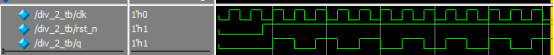二分频仿真结果图
（六分频）
module div_6(clk_out,clk,rst_n);
 output clk_out;
input clk;
input rst_n;

reg [1:0] cnt;
reg clk_out;

parameter N=6;

always @ (posedge clk or negedge rst_n)begin
if(rst_n==1'b0)
begin
cnt <= 0;
clk_out<= 0;
end
else  if(cnt==N/2-1)
begin
clk_out<=~clk_out;
cnt<=0;
end
else
cnt <= cnt + 1'b1;
end

endmodule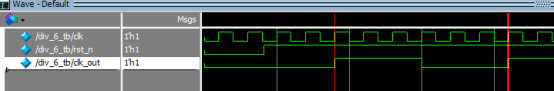六分频仿真结果图
2.奇分频（占空比50%）
实现奇数（N）分频，分别用上升沿计数到（N-1)/2，再计数到N-1；用下降沿计数到（N-1)/2，再计数到N-1，得到两个波形，然后把它们相或即可得到N分频。
（3分频）
module div_3 (q,clk,rst_n);
output q;
input rst_n;
input clk;
reg q1,q2;
reg [1:0] count1,count2;

always@(posedge clk or negedge rst_n)begin
if(rst_n==1'b0)
begin
q1<=1'b0;
count1<=2'b00;
end
else if(count1==0)
begin
q1<=~q1;
count1<=count1+1'b1;
end
else if(count1==1)
begin
q1=~q1;
count1<=count1+1'b1;
end
else
count1<=2'b00;
end

always@(negedge clk or negedge rst_n)begin
if(rst_n==1'b0)
begin
q2<=1'b0;
count2<=2'b00;
end
else if(count2==0)
begin
q2<=~q2;
count2<=count2+1'b1;
end
else if(count2==1'b1)
begin
q2=~q2;
count2<=count2+1'b1;
end
else
count2<=2'b00;
end
assign q=q1|q2;

endmodule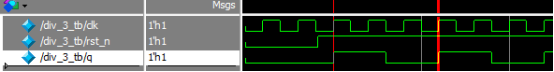3分频仿真图
（5分频）//5分频原理与3分频一样，只不过代码设计上简化了一点，不影响结果。
module test(
input clk,
input rst_n,
output q
);
reg [2:0] cnt;
reg q1, q2;

always@(posedge clk or negedge rst_n) begin
if(~rst_n) begin
cnt <= 0;
end
else if(cnt <3'd4) begin
cnt <= cnt + 1'b1;
end
else
cnt <= 0;

end

always@(posedge clk or negedge rst_n) begin
if(~rst_n) begin
q1 <= 1'b1;
end
else if(cnt == 3'd1) begin
q1 <= ~q1;
end
else if(cnt == 3'd4) begin
q1 <= ~q1;
end
else	q1 <= q1;
end

always@(negedge clk or negedge rst_n) begin
if(~rst_n) begin
q2 <= 1'b1;
end
else q2 <= q1;
end

assign q = q1 | q2;

endmodule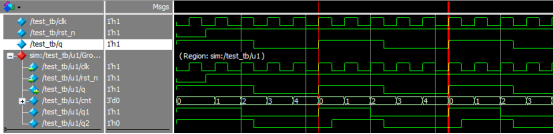5分频仿真结果图
3.占空比不为50%
在此拿三分频举例，分别给出1/3、2/3占空比例子。对于任意占空比首先要知道占空比是高电平与周期之比，然后通过计数产生高电平个数、取反，再计数到N-1取反，计数器置零。
（1）占空比2/3
module div3(clk,rst_n,q);
input			clk;
input			rst_n;
output	reg	q;

reg [1:0] cnt;

always@(posedge clk or negedge rst_n)begin
if(rst_n==1'b0)
begin
cnt<=2'd0;
q<=0;
end
else if(cnt==2'd0)
begin
q<=~q;
cnt<=cnt+1'b1;
end
else if(cnt==2'd2)
begin
q<=~q;
cnt<=2'd0;
end
else begin
q<=q;
cnt<=cnt+1'b1;
end
end

endmodule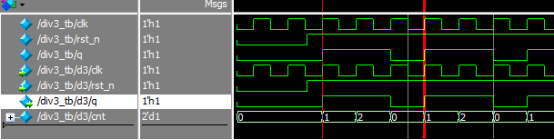占空比2/3仿真图
（2）占空比1/3
module div3(clk,rst_n,q);
input			clk;
input			rst_n;
output	reg	q;

reg [1:0] cnt;

always@(posedge clk or negedge rst_n)begin
if(rst_n==1'b0)
begin
cnt<=2'd0;
q<=0;
end
else if(cnt==2'd1)//代码仅此处计数值不同
begin
q<=~q;
cnt<=cnt+1'b1;
end
else if(cnt==2'd2)
begin
q<=~q;
cnt<=2'd0;
end
else begin
q<=q;
cnt<=cnt+1'b1;
end
end

endmodule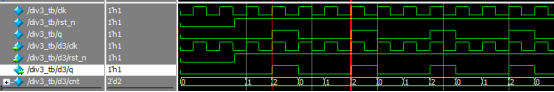占空比1/3
通过以上1/3、2/3可以知道对于其余不同占空比N分频应该可以有所理解。


展开全文verilog
• ## Verilog 分频器设计

千次阅读 多人点赞 2019-11-19 20:40:59
目录 1、偶分频 2、奇分频 3、任意分频和占空比 ...在数字电路中，使用 Verilog 生成不同频率的时钟和占空比是较为常见的一种设计，主要分为偶数分频，奇数分频，也可以任意进行分频和占空比的配置；...
目录

1、偶分频

2、奇分频

3、任意分频和占空比

在数字电路中，使用 Verilog 生成不同频率的时钟和占空比是较为常见的一种设计，主要分为偶数分频，奇数分频，也可以任意进行分频和占空比的配置；

1、偶分频

偶分频电路指的是分频系数为 2、4、6、8 ... 等偶数整数的分频电路，这种是比较简单的分频方式

例如下面 divider.v 中，对输入时钟进行6分频，即假设clk 为 50MHz ，分频后的时钟频率为 (50/6) MHz。程序如下：

//rtl
module clk_even_div(
input clk,
input rst_n,
output reg clk_div
);
parameter NUM_DIV = 6;
reg [3:0]cnt;

always @(posedge clk or negedge rst_n)
if(!rst_n) begin
cnt     <= 4'd0;
clk_div    <= 1'b0;
end
else if(cnt < NUM_DIV / 2 - 1) begin
cnt     <= cnt + 1'b1;
clk_div    <= clk_div;
end
else begin
cnt     <= 4'd0;
clk_div    <= ~clk_div;
end
endmodule

testbench为：

//testbench
timescale 1ns / 1ps
module testb_div_even;
// Inputs
reg clk;
reg rst_n;
// Outputs
wire clk_div;

// Instantiate the Unit Under Test (UUT)
clk_even_div uut (
.clk(clk),
.rst_n(rst_n),
.clk_div(clk_div)
);
always #10 clk = ~clk;

initial begin
// Initialize Inputs
clk = 0;
rst_n = 0;
// Wait 100 ns for global reset to finish
#100;
rst_n = 1;
end
endmodule



2、奇分频

由于奇分频需要保持分频后的时钟占空比为 50% ，所以不能像偶分频那样直接在分频系数的一半时使时钟信号翻转(高电平一半，低电平一半)。
接下来我们设计一个 5 分频的模块，设计思路如下：

采用计数器 cnt1 进行计数，在时钟上升沿进行加 1 操作，计数器的值为 0、1 时，输出时钟信号 clk_div 为高电平；计数器的值为2、3、4 时，输出时钟信号 clk_div 为低电平，计数到 5 时清零，从头开始计数。我们可以得到占空比为 40% 的波形 clk_div1。

采用计数器 cnt2 进行计数，在时钟下降沿进行加 1 操作，计数器的值为 0、1 时，输出时钟信号 clk_div 为高电平；计数器的值为2、3、4 时，输出时钟信号 clk_div 为低电平，计数到 5 时清零，从头开始计数。我们可以得到占空比为 40% 的波形 clk_div2。

clk_div1 和clk_div2 的上升沿到来时间相差半个输入周期，所以将这两个信号进行或操作，即可得到占空比为 50% 的5分频时钟。程序如下：

设计代码：

module clk_odd_div(
input clk,
input rst_n,
output clk_div
);

parameter NUM_DIV = 5;
reg[2:0] cnt1;
reg[2:0] cnt2;
reg    clk_div1, clk_div2;

always @(posedge clk or negedge rst_n)
if(!rst_n)
cnt1 <= 0;
else if(cnt1 < NUM_DIV - 1)
cnt1 <= cnt1 + 1'b1;
else
cnt1 <= 0;

always @(posedge clk or negedge rst_n)
if(!rst_n)
clk_div1 <= 1'b1;
else if(cnt1 < NUM_DIV / 2)
clk_div1 <= 1'b1;
else
clk_div1 <= 1'b0;

always @(negedge clk or negedge rst_n)
if(!rst_n)
cnt2 <= 0;
else if(cnt2 < NUM_DIV - 1)
cnt2 <= cnt2 + 1'b1;
else
cnt2 <= 0;

always @(negedge clk or negedge rst_n)
if(!rst_n)
clk_div2 <= 1'b1;
else if(cnt2 < NUM_DIV / 2)
clk_div2 <= 1'b1;
else
clk_div2 <= 1'b0;

assign clk_div = clk_div1 | clk_div2;

endmodule

testbench 为；

//testbench
timescale 1ns / 1ps
module testb_div_odd;
// Inputs
reg clk;
reg rst_n;
// Outputs
wire clk_div;

// Instantiate the Unit Under Test (UUT)
clk_odd_div uut (
.clk(clk),
.rst_n(rst_n),
.clk_div(clk_div)
);
always #10 clk = ~clk;

initial begin
// Initialize Inputs
clk = 0;
rst_n = 0;
// Wait 100 ns for global reset to finish
#100;
rst_n = 1;
end
endmodule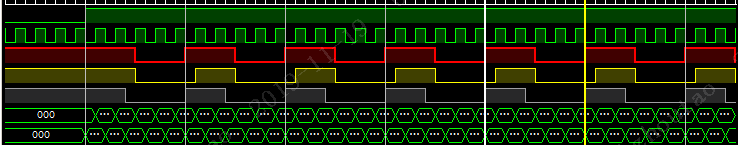绿色部分是输入的时钟，红色的部分是输出的 5 分频的时钟；

黄色和白色部分是 clk_div1 和 clk_div2

3、任意分频和占空比

获取的任意占空比和分频系数的方式是通过直接进行输入 clk 的值进行计数，在计数器到达某时刻，直接进行输出时钟的 0/1 翻转控制；

示例代码为：

module clk_div(
input clk,
input rst_n,
output reg clk_div
);

reg[5:0] cnt;
parameter MAX_CNT = 8;
parameter TOG_CNT = 2;

always @(posedge clk or negedge rst_n)
if(!rst_n)
cnt <= 1'b0;
else if(cnt < MAX_CNT)
cnt <= cnt + 1'b1;
else
cnt <= 1'b0;

always @(posedge clk or negedge rst_n)
if(!rst_n)
clk_div <= 1'b0;
else if(cnt < TOG_CNT)
clk_div <= 1'b1;
else
clk_div <= 1'b0;

testbench 为：

module clk_div;

// Inputs
reg clk;
reg rst_n;

// Outputs
wire clk_div;

// Instantiate the Unit Under Test (UUT)
clk_div uut (
.clk(clk),
.rst_n(rst_n),
.clk_div(clk_div)
);

always #10 clk = ~clk;

initial begin
// Initialize Inputs
clk = 0;
rst_n = 0;

// Wait 100 ns for global reset to finish
#100;

rst_n = 1;
end

endmodule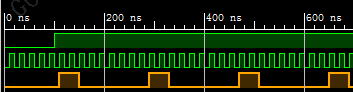黄色部分为分频出来的时钟；

参考：

https://www.cnblogs.com/zhangxianhe/p/11083208.html

展开全文• 偶分频：对于分频系数为10的分频器，本例的输入时钟系统50M时钟，输出为十分频时钟。设置一个3位的计数器，当计时寄存器到4（10/2-1）时，将输出分频信号取反即可得到10分频的输出。 module(clk,clk_out); input ...
偶分频：对于分频系数为10的分频器，本例的输入时钟系统50M时钟，输出为十分频时钟。设置一个3位的计数器，当计时寄存器到4（10/2-1）时，将输出分频信号取反即可得到10分频的输出。

module(clk,clk_out);
input clk;//输入时钟频率
output clk_out;//输出输出时钟频率
reg clk_out;
reg [2：0] cnt;//计数器,假设分频系数为10

always @(clk);
if(cnt = 3'b1000)begin
clk_out <= ~clk_out;
cnt <= 'b0;
end
else begin
cnt <= cnt + 'b1;
end

奇分频：实现奇数分频原理是分别用上升沿计数到N/2+1，时钟进行翻转，再计数到N/2输出out_clk1，再用下降沿计数到N/2+1，时钟进行翻转，再计数到N/2输出out_clk2，将out_clk1和out_clk2相或即可。可以通过修改N的值和计数器的位宽来实现其他奇数分频。

always @(posedge clk or negedge rst_n)
//上升沿输出out_clk1
begin
if(!rst_n)
begin
out_clk1<=0;
cnt_1<=0;
end
else
begin
if(out_clk1 == 0)
begin
if(cnt_1 == N/2+1)
begin
out_clk1<=~out_clk1;
cnt_1 <= 0;
end
else
cnt_1 <= cnt_1+1;
end
else
if(cnt_1 == N/2)
begin
out_clk1<=~out_clk1;
cnt_1 <= 0;
end
else
cnt_1 <= cnt_1+1;
end
end

always @(negedge clk or negedge rst_n)
//下降沿输出out_clk2
begin
if(!rst_n)
begin
out_clk2 <= 0;
cnt_2 <= 0;
end
else
begin
if(out_clk2 == 0)
begin
if(cnt_2 == N/2+1)
begin
out_clk2 <= ~out_clk2;
cnt_2 <= 0;
end
else
cnt_2 <= cnt_2+1;
end
else
if(cnt_2 == N/2)
begin
out_clk2 <= ~out_clk2;
cnt_2 <= 0;
end
else
cnt_2 <= cnt_2+1;
end
end

assign out_clk = out_clk1 | out_clk2;


展开全文• 这是一个基于verilog语言的分频器的设计的代码，在设置的位宽范围以内任意系数的分频器均可以采用本代码。当然，讲寄存器的位宽设置更高，可以继续增加分频系数
• D触发器实现分频器 对于分频器的实现，我们最常用的方法就是通过计数器来实现，具体的实现可以看前面的文章，但是通过D触发器实现却很少遇到，我将通过这篇文章讲解D触发器实现分频器，这里以9分频为例。 我们要实现...
• 1.简介 1.在数字电路设计的过程中，常常需要对较高频率的时钟信号进行分频操作，从而得到较低频率的时钟信号。 一个硬件电路，如果时钟...2.分频器：完成对时钟信号的2分频，4分频，8分频和16分频 2.分频器设计 ...
• 数字分频器的设计，包括VerilogHDL设计实现，以及仿真波形
• 频器，就是将特定频率的信号，通过计数分频的方式，得到自己需要的频率的信号。比如假如你的系统时钟是50Mhz,而你需要得到一个25Mhz的信号，则可以设计一个二分频模块对系统时钟进行分频获得。设计方法很简单，...fpga
• 尤其是蓝绿光单激光具有较高的相干性,可广泛应用于高分辨率光谱、原子冷却和俘获、量子光学等领域,吸引了国内外学者的极大关注,发展十分迅速。本文介绍了实现蓝绿光单激光的几种关键技术——二次谐波产生(SHG)...
• 频器，顾名思义，就是将一个波形，分成具有若干占空比的波。占空比是指在一个脉冲循环内，通电时间相对于总时间所占的比例。占空比(Duty Ratio)在电信领域中有如下含义:例如:脉冲宽度1μs，信号周期4μs的脉冲序列...verilog FPGA
• 频器是指使输出信号频率为输入信号频率整数分之一的电子... 早期的分频器多为正弦分频器，随着数字集成电路的发展，脉冲分频器（又称数字分频器）逐渐取代了正弦分频器。 下面以Verilog HDL 语言为基础介绍占空比...
• 在数字电路中，常需要对较高频率的... 下面我们介绍分频器的VHDL描述，在源代码中完成对时钟信号CLK的2分频，4分频，8分频，16分频。LIBRARY IEEE;USE IEEE.STD_LOGIC_1164.ALL;USE IEEE.STD_LOGIC_ARITH.ALL;USE I...
• 在用HDL实现分频器的时候，主要通过写计数器计数时钟的上升/下降沿来实现。偶数倍分频十分容易实现在此不加赘述，奇数倍分频需要通过计数器生成两个波形然后进行异或操作实现奇数倍分频。 以下给出5倍分频、10倍分频...FPGA verilog testbench
• 通常实现偶数的分频比较容易，以十分频为例: always @( posedge clk or posedge reset)  if(reset)  begin  k;  clk_10;  end  else  if(k==4)  begin  k;  clk_10~clk_10;  end   else  k; 　二...
• 硬件设计的电路，是用集成计数器74LS161构成可编程分频器
• 1. 偶数分频器 偶数分频器的实现较为简单，用计数器即可实现 // 偶数分频器示例，20分频即N=10，占空比50% module Fre_div_even( input clk, input rst_n, input [3:0] N, // N = 分频倍数/2 output reg clk...Verilog FPGA 逻辑
• ## Verilog实现分频器

千次阅读 2016-07-21 13:36:03
module pregate(clk,gateout); input clk; //输入标准时钟 output gateout;//输出预设闸门信号 reg gateout,gatebuf; reg [19:0] cnt;//计数器 always @(posedge clk) ...//计数值越大，闸门时间越长 ...FPGA verilog
• 一、偶数分频器 100Mhz二分频后为50Mhz. 偶数分频实现比较简单，假设为N（偶数）分频，只需计数到N/2 -1 然后时钟翻转，计数器清零。 Example 使用Verilog语言设计4分频电路。 module divide_2 ( input clk, ...verilog
• CMOS射频收发的无生产线半导体制造商Quorum Systems宣布，已开始批量生产Sereno QS1000四GSM/GPRS/EDGE收发。 　Quorum Systems总裁兼CEO，BernardXavier说：“在任何一家新兴半导体公司的发展生涯中，种......